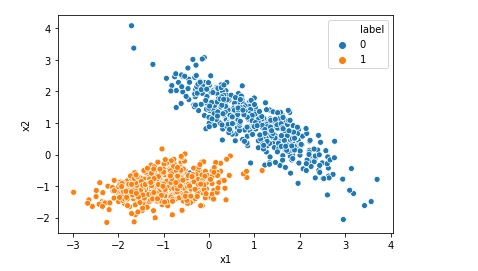# Top 5 differences between Python and Golang programming language

In this post we are going to discuss top 5 differences between Python and Golang programming language. https://youtu.be/9-jaw-nB3bE Python Vs Golang Top 5 differences Python is an interpreted language while golang is a compiled language.As python is interpreted language, The python code has to be converted into a machine-readable form, by a program called interpreter, … Continue reading Top 5 differences between Python and Golang programming language# Thonny is a Simple Python IDE for Beginners

Thonny is a Simple Python IDE for Beginners is a short video to discuss the top 10 features of Thonny. Although Thonny is very suitable for beginners, it has several useful features that make it a good IDE for full-fledged Python development. Thonny is included by default in the Raspberry Pi OS https://youtu.be/7foELT_oljI Thonny is … Continue reading Thonny is a Simple Python IDE for Beginners# Top Ten Reasons for NOT learning Python Programming

This post is not to discourage any one :)The aim of this post is to discuss, if you have started learning or planning to learn Python programming, are you on the right path?I don't think that there's a lot of good uses NOT to learn something, however, the argument is "could my time be better … Continue reading Top Ten Reasons for NOT learning Python Programming

# Difference Between Batch, Mini-Batch and Stochastic Gradient Descent

Gradient Descent is one the key algorithm used in Machine Learning. While training machine learning model, we require an algorithm to minimize the value of loss function. Gradient Descent is one of the optimization algorithm , that is used to minimize the loss. There are mainly three types of Gradient Descent algorithm1. Batch Gradient DescentBatch … Continue reading Difference Between Batch, Mini-Batch and Stochastic Gradient Descent

# How to write command-line arguments using argparse in python

If you want to run a .py python file from command line and you also want to pass the argument using command line you can use argparse library. This can be done as below: import argparse # Command Line arguments argp = argparse.ArgumentParser() argp.add_argument('--my_var', dest="my_var", action="store", type=int, default=5) params = argp.parse_args() myvar= params.my_var print(myvar) Then … Continue reading How to write command-line arguments using argparse in python# Handling missing data in pandas data frame python

In this post we are going to discuss how to handle missing data from a pandas data frame. Find total number of missing data in the data frame missing_total = df.isnull().sum().sum() Find number of missing data in each column in a data frame missing_per_column = df.isnull().sum() Investigate patterns in the amount of missing data in … Continue reading Handling missing data in pandas data frame python

# int object is not iterable Error

If your code is like below count = 100 for number in count: This will give you error like.. int object is not iterable. In Python, the thing you pass to a for statement needs to be some kind of iterable object. The variable count here is a number which is not iterable. You should … Continue reading int object is not iterable Error# Random Forest Classification in Python in 10 Lines

Random Forest algorithm is like an ensemble algorithm made of Decision Trees, which comprises more than one decision tree to create a model. It creates more than one tree like conditional control statements to create its model hence it is named as Random Forest. Random Forest machine learning algorithm can be used to solve both … Continue reading Random Forest Classification in Python in 10 Lines# Random Forest Regression in Python in 10 Lines

Random Forest algorithm is like Decision Tree, which comprises more than one decision tree to create a model. Random Forest algorithm is an ensemble method. It creates more than one tree like conditional control statements to create its model hence it is named as Random Forest. Random Forest machine learning algorithm can be used to … Continue reading Random Forest Regression in Python in 10 Lines# Implementing Logistic Regression in 10 lines in Python

Logistic Regression is one of the most popular Machine Learning algorithm used for the classification problems. It should be noted that though there is a regression word in the name of the algorithm Logistic Regression, it is used for classification problems. A use case of Logistic regression could be, based on the symptoms for a disease that a patient has Logistic … Continue reading Implementing Logistic Regression in 10 lines in Python